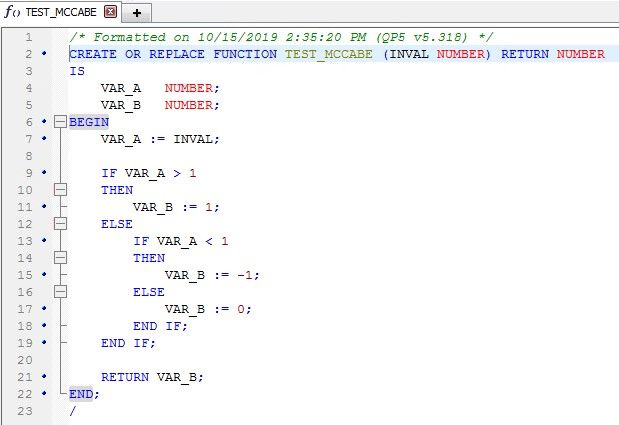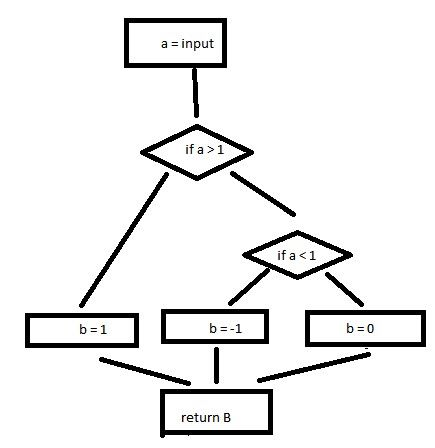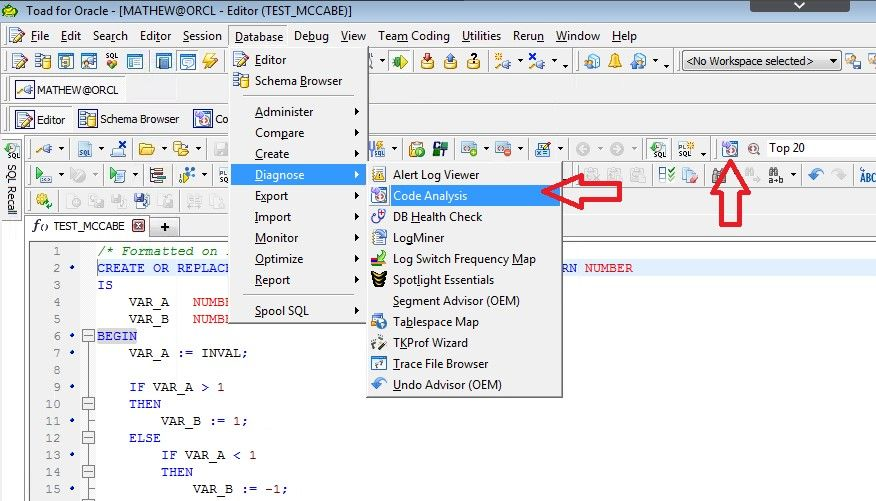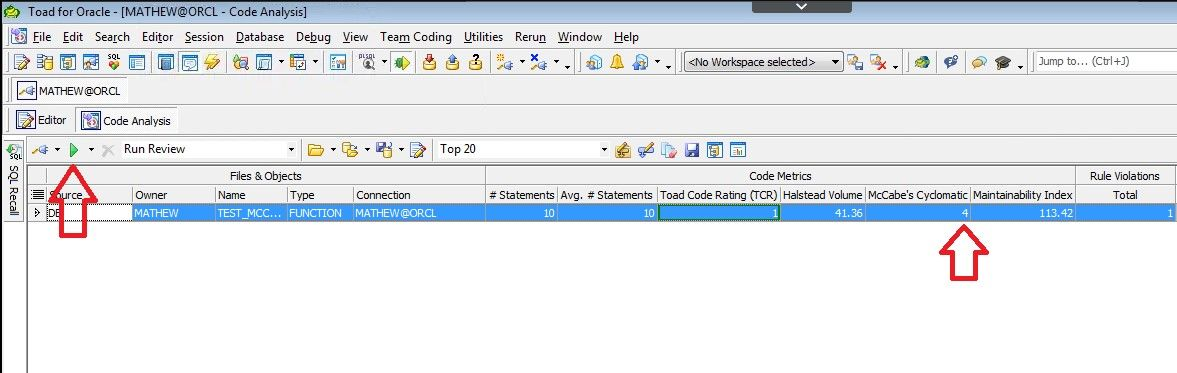## What is McCabe Cyclomatic Complexity and How Does it Affect Code Testing?

Earlier this year I did a webinar on the Toad for Oracle Code Analysis feature and I demonstrated how Code Analysis takes a look at your PL/SQL code and automatically runs a series of algorithms to score your code using common computer science metrics. One of the metrics it provides is McCabe’s Cyclomatic Complexity.

## What is McCabe Cyclomatic Complexity?

This score helps you determine how complex your code is. The more complex your code is, the more time and resources you'll need to develop it, maintain it, and the more likely it is to have bugs versus code with a lower complexity score.

A higher complexity indicates more pathways within the code. That means if you want full code coverage testing, then you'll have to design and run more tests cases. A score of 10 or less is considered a pretty simple and easy to maintain, 11-20 indicates a somewhat complex program, then 21-50 being a very complex program, and anything over 50 being so complex that it is untestable. Can you imagine how tough it is to come up with 50 test cases, then document it, and then have to rerun all of them if the code ever changes?

## How is McCabe Cyclomatic Complexity calculated?

Let's take a look at how this value is calculated. Take the following code for example:The idea of this stored procedure is that given an input value as a number, we will store that value as a variable A. If the A is representing any positive number, then we'll store B with the value of 1. If A is any negative value, then we'll store B as -1. And finally, if A is not greater than 1 and not less than 1, then it is equal to 0, then we'll store B with a value of 0 as well.

This code is simple enough where just looking at it you may quickly see that there are three possible paths depending on if A is positive, negative or zero. Although there are three possible outcomes, B being 1, -1, or 0, there is really only one "out" and that is assigning a value to B and returning it to the caller.

If we were to try to visually represent this in a flow chart, you may come up with something like this (please excuse my MS Paint skills):In this diagram, a chunk of code is represented by a shape. We will represent lines of code that execute in succession as a rectangle such as creating the variables A and B, then assigning the input value to A. If the line of code has a predicate, such as "if" or "while" statements, they have more than one pathway, so those are represented as a diamond. The flow from one shape to another is a line, or an edge. Lastly, there is only one way out of this code and that is returning the value of B to the original caller.

So, in this diagram I have 7 shapes and 8 edges. I also have 1 exit point which is after print B.

McCabe's Cyclomatic can be broken down into the following formula.

M = E – S + 2*P

Where M is the complexity, E is the number of Edges, and P is the number of program exits points. Which results in:

8 – 7 + 2 * 1 = 3.

The three paths, just as we predicted earlier. There are different ways to draw the flow diagram, but it usually results in the same complexity value in the end. For example, adding or removing a rectangle shape would also add or remove an edge as well.

I could have combined the bottom 4 rectangles into one rectangle (setting the value for B, then returning B) thus reducing the number of rectangles by 3, but that would also reduce the number of edges by 3.  Now it is 4 shapes total, 5 edges which still results in complexity of 3:

5 – 4 + 2 * 1 = 3.

This just shows that you can draw the diagram differently, but if you follow the rules, the calculations will come out the same.

## Why does it matter?

In this example the complexity value is 3, then you need to have at least three test cases in order to be sure each possible path in this code has been tested.

The more variables, more predicates, and more exit points in your code, the more overall complex it is. I'm not saying that code with a high McCabe's Cyclomatic value is bad. It's just saying that it is complex, harder to maintain, and you will need more test cases in order to ensure code coverage, but sometimes it's the only way to code what you're trying to do.

It forces the developer to ask, is there a better way to do this? If the answer is no, then that's fine. If the answer is yes, awesome, take that more optimum approach.

Now that you understand how it's calculated, are you really going to sit down and draw out diagrams for all your code? Not likely. So here's a reminder on how to do it in Toad for Oracle.

## How to use Toad for Oracle to calculate McCabe Cyclomatic Complexity

Open your code in the Editor window. Then open Code Analysis by going to the menu Database | Diagnose | Code Analysis. You can also click on the icon highlighted with the other red arrow.On the next screen, you can add more database objects if you want. Click on Analyze Code. See the value shown here.You may notice the value shown by Toad is not exactly the same as one you may come up with when using a diagram or using the formula. Toad does a simple calculation by just adding up the number of pathways in your code, but it’s still a very helpful metric to have.

Now that you know the McCabe Cyclomatic metric is 4, we should create at least 4 unit tests to cover each pathway of the code. Some may think that creating 4 test cases is really time consuming, but it's actually really simple in Toad for Oracle Developer Edition's Code Tester feature. Also, the next release of Toad for Oracle will have a renewed focused on unit testing with its new, built in, utPLSQL support. Stay tuned for a future blog post where I go into the details of unit testing in Code Tester and utPLSQL.

With the combination of Code Analysis and unit testing, you’ll ensure your code is easy to maintain.

## Try Toad for Oracle now:

Try Toad for Oracle free for 30 days.